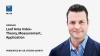### Keep current with knowledge base articles from METER Environment

DR. GAYLON S. CAMPBELL

The radiation reaching the probe of the ACCUPAR LP-80 can come directly from the solar beam or be scattered from the sky or clouds. These two sources are affected differently by canopy architecture and must, therefore, be treated separately in the computation of leaf area index (LAI) from canopy transmission measurements. The information needed to make the computation is the beam fraction or ratio of radiation that comes directly from the solar beam to the total radiation (beam plus scattered or diffuse PAR) incident on the probe.

## No more shading the probe

The previous version of the ACCUPAR required the user to measure beam fraction by shading the probe. The LP-80 computes it using measurements it has available. The method used is modified from one published by Spitters et al. (1986) to find beam fraction for total radiation. They correlated beam fraction with the ratio of measured total global radiation to potential radiation on a horizontal surface outside the earth’s atmosphere.

The above-canopy measurement of PAR from the LP-80 is the total global PAR value. Since latitude and time of day are known, the potential PAR (PAR on a horizontal surface outside the earth’s atmosphere) can be computed. The ratio of these two measurements is related to the fraction of the total PAR in the solar beam just as Spitters et al. did. The procedure in the LP-80 is as follows:

1. Compute r, the fraction of potential PAR that reaches the probe. This is the PAR “solar constant” times the cosine of the zenith angle, divided into above-canopy PAR reading. We assume the PAR “solar constant” to be 2550 µmol/m2/s.
2. An r value of 0.82 or above is set to 0.82, a clear sky; a value of 0.2 or below is set to 0.2, a fully diffuse sky.
3. The fraction r is used in the following empirical polynomial, derived from data, to compute beam fraction:The macro for doing this calculation is given in the Appendix.

## LP-80 theory saves time and effort

This approach is likely to be less accurate than a direct measurement of fb, if that measurement were done very carefully, but it is difficult to do direct measurements of fb on a routine basis while one is trying to measure canopy interception or LAI. In fact, the errors introduced by the approximate method used in the LP-80 are typically small compared to other measurements’ errors. The following graph shows the error in LAI as a function of error in estimating beam fraction, assuming a constant beam fraction of 0.4 was used for all LAI calculations.

This error is independent of LAI. The calculations are for a zenith angle of 30 degrees. Larger zenith angles have smaller errors. The graph shows that the error in LAI is always smaller than ± 20%. For a 10% error in beam fraction, the error in LAI is around 2%. It is difficult to know how large errors could be in the LP-80 method for computing beam fraction since that depends on conditions, but they are likely in the range of 10 to 20%. The error this introduces into the LAI calculation is therefore in the range of 2 to 4%, which is considerably smaller than uncertainties from spatial variability in the measurement of LAI.Figure 1. The error in LAI as a function of error in estimating beam fraction, assuming a constant beam fraction of 0.4 was used for all LAI calculations

### Reference

Spitters, C. J. T., H. A. J. M. Toussaint, and J. Goudriaan. “Separating the diffuse and direct component of global radiation and its implications for modeling canopy photosynthesis part I. components of incoming radiation.” Agricultural and Forest Meteorology 38, no. 1-3 (1986): 217-229. Article link.

### Appendix: Visual BASIC macro for computing beam fraction

```Function BeamFraction(Zenith As Single, PAR As Single) As Single
Const pi = 3.14159
Dim r As Single, b As Single

Zenith = Zenith * pi / 180
If Zenith > 1.5 Then
b = 0#     'nighttime
Else
r = PAR / (2550# * Cos(Zenith)) '600 w/m2 * 4.25 umol/w/m2 (.235 MJ/mol)(600 is potential PAR)
If r > 0.82 Then r = 0.82
If r < 0.2 Then r = 0.2
b = 48.57 + r * (-59.024 + r * 24.835)
b = 1.395 + r * (-14.43 + r * b)
End If
BeamFraction = b
End Function
```

## Questions?

Explore questions and ideas with a canopy expert. METER scientists have over 100 years combined experience measuring the soil-plant-atmosphere continuum.  Learn more about canopy measurement in the video below. Dr. Steve Garrity discusses Leaf Area Index (LAI).  Topics covered include the theory behind the measurement, direct and indirect methods, variability among those methods, things to consider when choosing a method, and applications of LAI.